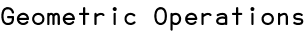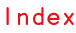RSS

Scriptographer offers several ways to create new paths out of two overlapping paths.

## Intersection

path.intersect(path) returns a new path, the shape of which is the intersection of the two paths: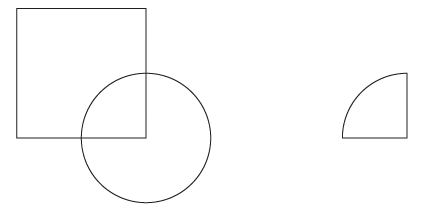```var square = new Path.Rectangle([0, 0], [50, 50]);
var circle = new Path.Circle(square.bounds.bottomRight, 25);

// Ihe intersection of the paths:
var intersection = square.intersect(circle);

// Move the resulting path 100pt to the right:
intersection.position += [100, 0];
```

## Union

path.unite(path) returns a new path, the shape of which is the union of the two paths: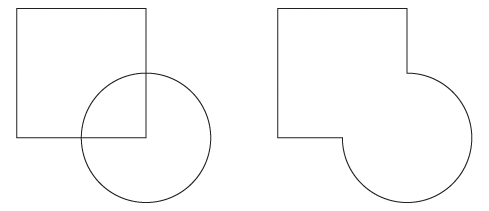```var square = new Path.Rectangle([0, 0], [50, 50]);
var circle = new Path.Circle(square.bounds.bottomRight, 25);

// Ihe union of the paths:
var union = square.unite(circle);

// Move the resulting path 100pt to the right:
union.position += [100, 0];
```

## Exclusion

path.exclude(path) returns a new path, the shape of which is the exclusion of the path passed to the exclude function from the path that the exclude function is called on: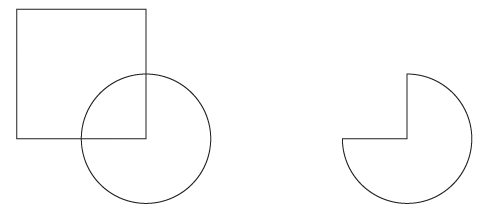```var square = new Path.Rectangle([0, 0], [50, 50]);
var circle = new Path.Circle(square.bounds.bottomRight, 25);

// Ihe exclusion of the paths:
var exclusion = square.exclude(circle);

// Move the resulting path 100pt to the right:
exclusion.position += [100, 0];
```